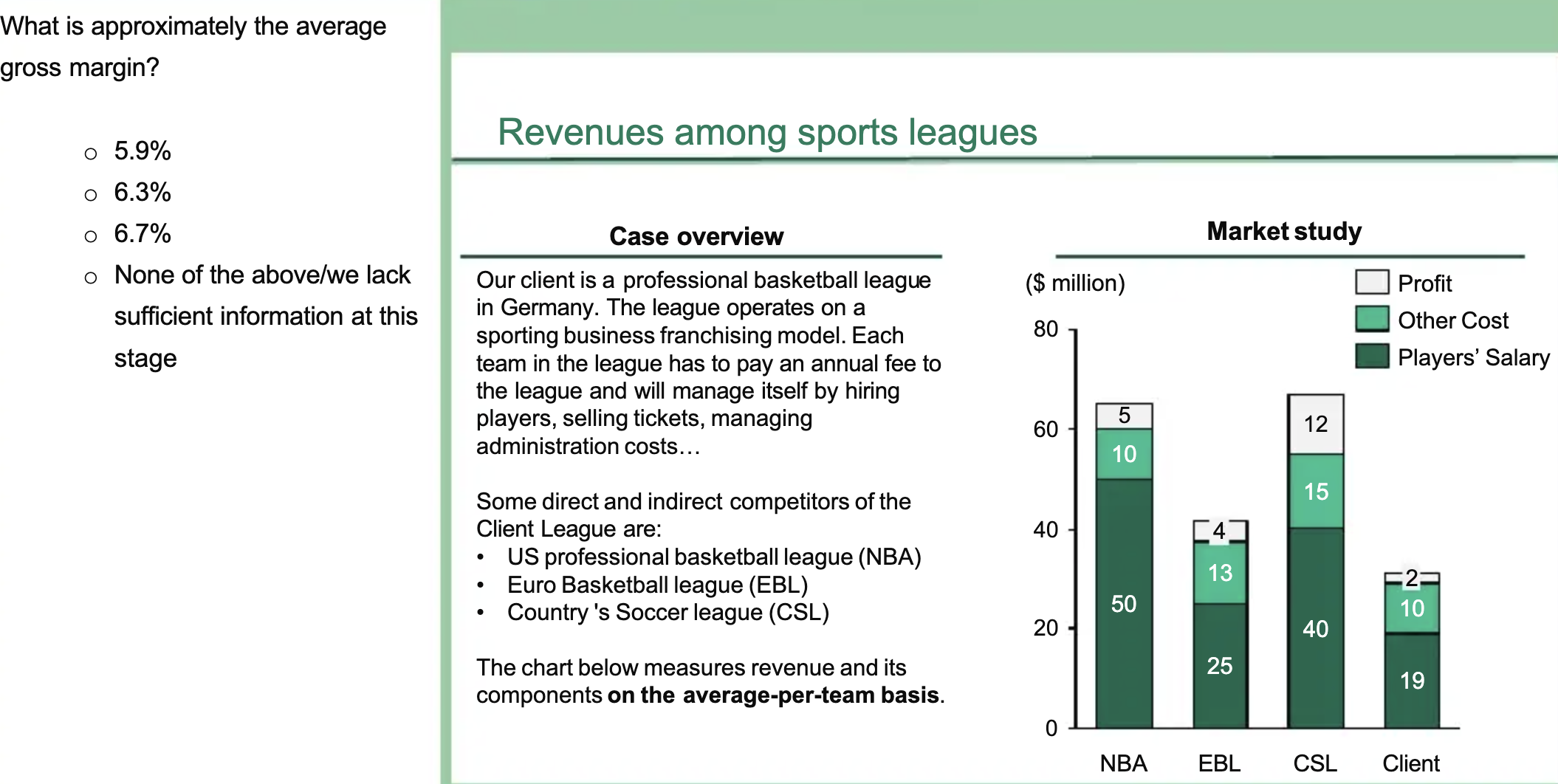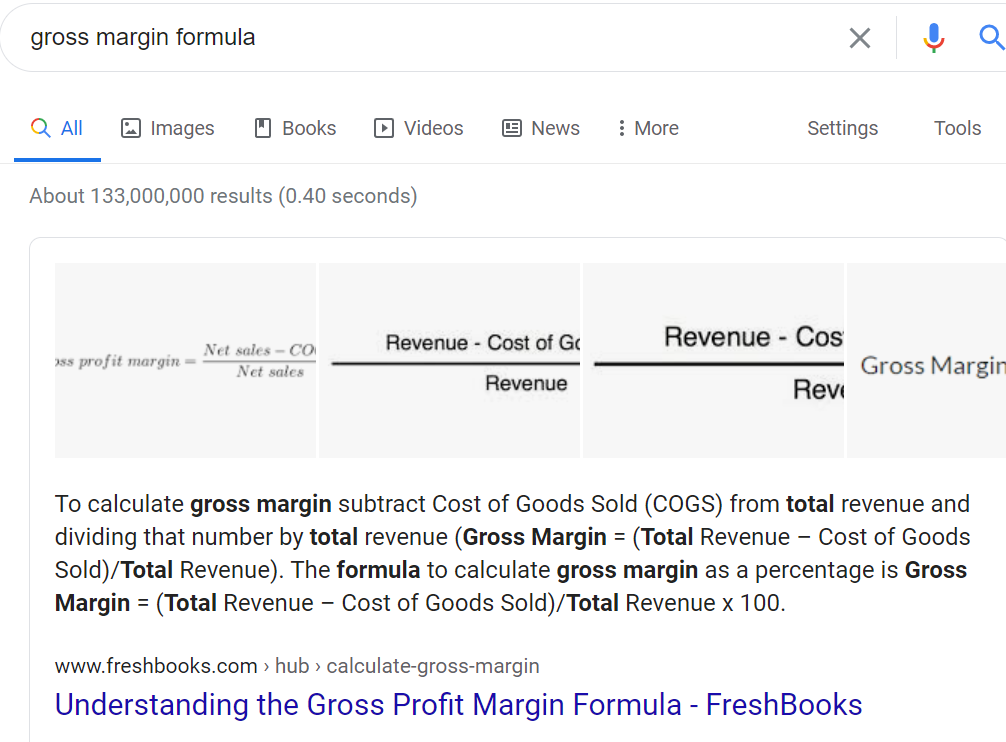# BCG Potential Test

BCG BCG Math BCG Potential Test case math GMAT MBB PST
New answer on Oct 15, 2020
3.2 k Views

Hello,Does anyone please know how to tackle the attached math word problem?(edited)

• Date ascending
• Date descending

To calculate gross margin subtract Cost from total revenue and divide that number by total revenue.

In other words, divide the profit by the total revenue. So in the case of the NBA it would be 5/65, and for EBL 4/42 and so on.

So just add up all the profits (5+4+12+2=23) and all the revenues (65+42+67+31=205) and this would be 23/205, or approx 9%. None of the above.

PS I believe that this formula is preferable to the other because it makes few sense to sum single margin percentages and divide the total by four, because I wouldn't weigh the margins by the revenue and this wouldn't provide me with a useful business information.

Thank you Stefano

Get the gross margin formula and calculate it for each individual player. Then, average it out (i.e. add them and divide by 4)

NBA: 5/65 = 7.7%

EBL: 4/42 = 9.5%

CSL: 12/67 = 17.9%

Client: 2/31 = 6.5%

(8.3+10.53+21.82+6.89)/4 = 10.4%(edited)

Thanks Ian

Hi there,

The gross margin is equivalent to (profit)/(revenue) and in this situation it's (profit)/(profit+costs).

This way you can calculate the exact result and choose the adequate answer.

I hope this helps!

Mehdi